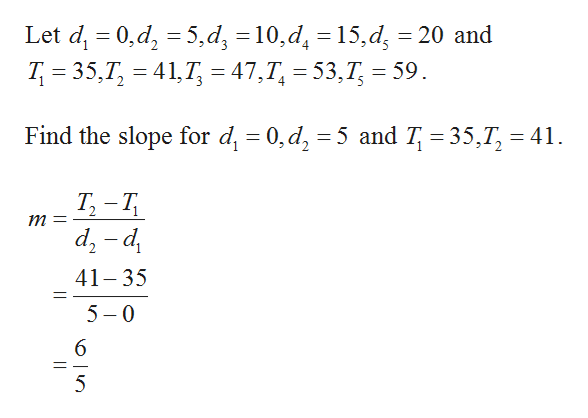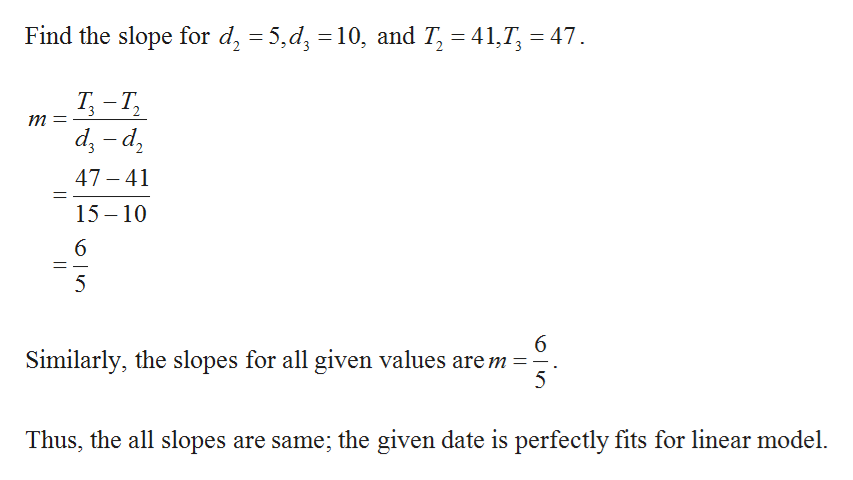# During a certain flu epidemic, the total number T of patients diagnosed with the flu after d days is given in the table below.d05101520T3541475359(a) Check differences to show that these are linear data.T is a not linear function or T is a linear function?   (b) What is the practical meaning of the slope?The slope represents the total number of cases each day orThe slope represents the number of new cases per day  ?

Question

During a certain flu epidemic, the total number T of patients diagnosed with the flu after d days is given in the table below.

 d T 0 5 10 15 20 35 41 47 53 59
(a) Check differences to show that these are linear data.
T is a not linear function or T is a linear function?

(b) What is the practical meaning of the slope?
The slope represents the total number of cases each day or
The slope represents the number of new cases per day  ?
check_circle

Step 1

(a)

It is known that, any set of data is linear then the slope is constant.help_outlineImage TranscriptioncloseLet d 0,d, 5,d, 10,d = 15,d, = 20 and T 35,7 417= 47,T, = 53,7T, = 59. Find the slope for di 0,d, 5 and T, 35,T 41 Т, — Т, т 3 p-p 41 35 5 0 6 fullscreen
Step 2help_outlineImage TranscriptioncloseFind the slope for d, 5,d 10, and T, 41, 47 Т -Т, Т, т 3 2 р- р 47-41 1 15 10 5 6 Similarly, the slopes for all given values are m 5 Thus, the all slopes are same; the given date is perfectly fits for linear model. fullscreen

### Want to see the full answer?

See Solution

#### Want to see this answer and more?

Solutions are written by subject experts who are available 24/7. Questions are typically answered within 1 hour.*

See Solution
*Response times may vary by subject and question.
Tagged in

### Algebra